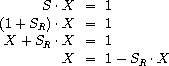# Invert-unit-series procedure

Let `S` be a power series (exercise 3.59 ) whose constant term is `1` . Suppose we want to find the power series `1/S` , that is, the series `X` such that `S · X = 1` . Write `S = 1 + SR` where `SR` is the part of `S` after the constant term. Then we can solve for `X` as follows:In other words, `X` is the power series whose constant term is `1` and whose higher-order terms are given by the negative of `SR` times `X` . Use this idea to write a procedure `invert-unit-series` that computes `1/S` for a power series `S` with constant term `1` . You will need to use `mul-series` from exercise 3.60